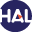Home page > 09. Volume 6 N°1 (2009) > Error estimates for a finite volume method for the Laplace equation in (...)

Error estimates for a finite volume method for the Laplace equation in dimension one through discrete Green functions

Sunday 6 December 2009,

Full Title:

Error estimates for a finite volume method for the Laplace equation in dimension one through discrete Green functions

Abstract: The cell-centered finite volume approximation of the Laplace equation in dimension one is considered. An exact expression of the error between the exact and numerical solutions is derived through the use of continuous and discrete Green functions. This allows to discuss convergence of the method in the L∞ and L2 norms with respect to the choice of the control points in the cells and with respect to the regularity of the data. Well-known second-order convergence results are recovered if those control points are properly chosen and if the data belongs to H 1 . Counterexamples are constructed to show that second-order may be lost if these conditions are not met.

Paper presented by: Frédéric PascalVoir sur HAL : https://hal.archives-ouvertes.fr/cea-00391590